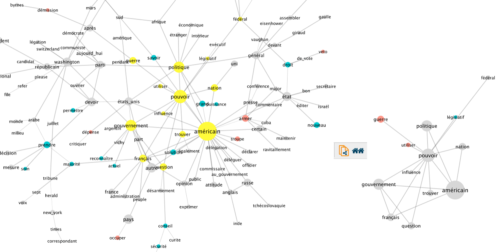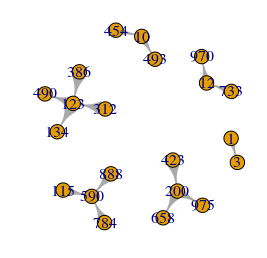# Create a subgraph from the neighborhood of specific vertices in igraphMany user of igraph for R expect the functions ego() and make_ego_graph() , that take a list of vertices as input, to generate a new graph composed of the neighbors of these vertices. Unfortunately, these functions do no such thing. They generate a list of igraph.vs objects, which cannot be further treated as an igraph object. This must have some historic reason but is currently unpractical, considering that neigborhood-based subgraph genaration is a feature so common that a software like Cytoscape even dedicates one of the few buttons of its GUI to its use.

There is a workaround, though. It lies in the combination of unlist()  and the function induced_subgraph() , also part of the igraph package for R. Here is a short example of use:

```library(igraph)
# generate a random graph with named vertices
g <- sample_gnp(1000,0.001,directed = TRUE)
V(g)\$name <- as_ids(V(g))

# make a list of the names of the nodes of interest
nodes_of_interest <- c("1","200","123","590","10","12")

# select the nodes having these names
selnodes <- V(g)[name %in% nodes_of_interest]
# get their network neighborhood
selegoV <- ego(g, order=1, nodes = selnodes, mode = "all", mindist = 0)

# turn the returned list of igraph.vs objects into a graph
selegoG <- induced_subgraph(g,unlist(selegoV))

# plot the subgraph
plot(selegoG,vertex.label=V(selegoG)\$name)```•
•
•
•
•